# Puzzle | Maximum number of Kings on Chessboard without under check

• Difficulty Level : Easy
• Last Updated : 27 May, 2018

Prerequisite – The Pigeonhole Principle
Problem statement – Given a 8×8 chessboard, figure out the maximum number of kings that can be placed on the chessboard so that no two kings attack each other, i.e., none of the kings is under check. A king can move only one step at a time any direction on the chessboard (horizontally, vertically and diagonally).

Explanation –
According to Pigeonhole principle if we have n+1 pigeons and we have n pigeon holes (or rather cages) then we must have one hole (or cage) with more than one pigeon.

For example if I had 6 balls and 5 boxes. I put all the balls in the boxes (any box can have any number of balls). Then the pigeonhole principle states that no matter how you put the balls inside the boxes, there will atleast be one box always that has more than one ball. It’s a very intuitive principle in mathematics and yet the problems associated with the principle are so hard to comprehend.

So, here’s the chessboard. Does this problem have anything to do with the pigeonhole principle?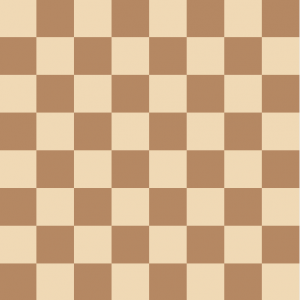Figure – 8×8 Chessboard

The answer to this problem is 16. But how do we arrive at this solution. The thing to note in this puzzle is that whenever we have two kings within a 2×2 square, they are always under check :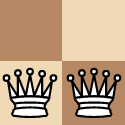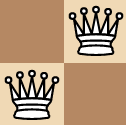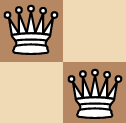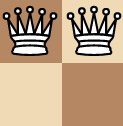No matter how we place 2 kings within a 2×2 chessboard we will always have them under check. By this observation, we can easily conclude that we can have at max one king within a 2×2 square.

We can imagine a 2×2 square as a hole (cage) for our pigeon i.e. kings. So, a 2×2 square occupies 4 sq units of area. The total area for the square chess board is 64 square units (assuming the size of chessboard to be 8 units × 8 units). So, we have 64/4 = 16 cages or holes in this scenario.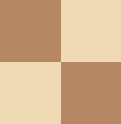We can easily place one king each in these big squares of 2×2. After 16 kings are completed , we would always have a scenario where two kings are placed in a 2×2 square (by Pigeon Hole Principle). This violates our initial condition and hence we can have at max 16 kings which satisfy the above condition.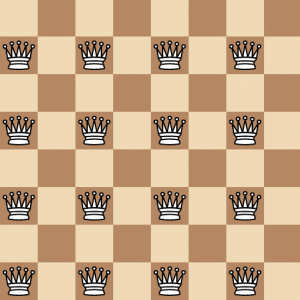Figure – Possible solution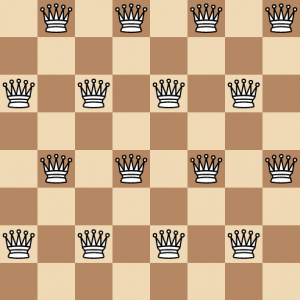Figure – Another possible solution

Generalized Formula –
Even for a n×n square the cage size (2×2 square) remains the same and we can easily say that whenever you exceed the number of cages possible you will definitely have two kings within a 2×2 square. Hence the maximum number of cages is given by:This article is contributed by Ankit Jain . If you like GeeksforGeeks and would like to contribute, you can also write an article using contribute.geeksforgeeks.org or mail your article to contribute@geeksforgeeks.org. See your article appearing on the GeeksforGeeks main page and help other Geeks.

Please write comments if you find anything incorrect, or you want to share more information about the topic discussed above.

My Personal Notes arrow_drop_up